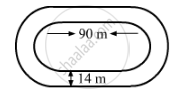Share

# The Inside Perimeter of a Running Track (Shown in Fig. 20.24) is 400 M. the Length of Each of the Straight Portion is 90 M - Mathematics

Course
ConceptIntroduction of Mensuration

#### Question

The inside perimeter of a running track (shown in Fig. 20.24) is 400 m. The length of each of the straight portion is 90 m and the ends are semi-circles. If track is everywhere 14 m wide, find the area of the track. Also, find the length of the outer running track.#### SolutionIt is given that the inside perimeter of the running track is 400 m . It means the length of the inner track is 400 m .
Let r be the radius of the inner semicircles .
Observe: Perimeter of the inner track = Length of two straight portions of 90 m + Length of two semicircles
∴ 400 = (2 x 90) + (2 x Perimiter of a semicircle)
$400 = 180 + (2 \times \frac{22}{7} \times r)$
$400 - 180 = (\frac{44}{7} \times r)$
$\frac{44}{7} \times r = 220$
$r = \frac{220 \times 7}{44} = 35 m$
∴ Width of the inner track = 2r = 2 x 35 = 70 m
Since the track is 14 m wide at all places, so the width of the outer track: 70 + (2 x 14) = 98 m
∴ Radius of the outer track semicircles $= \frac{98}{2} = 49 m$
Area of the outer track = (Area of the rectangular portion with sides 90 m and 98 m) + (2 x Area of two semicircles with radius 49 m)
$= (98 \times 90) + (2 \times \frac{1}{2} \times \frac{22}{7} \times {49}^2 )$
$= (8820) + (7546)$
$= 16366 m^2$
And, area of the inner track = (Area of the rectangular portion with sides 90 m and 70 m) + (2 x Area of the semicircle with radius 35 m)
$= (70 \times 90) + (2 \times \frac{1}{2} \times \frac{22}{7} \times {35}^2 )$
$= (6300) + (3850)$
$= 10150 m^2$
∴ Area of the running track = Area of the outer track - Area of the inner track
$= 16366 - 10150$
$= 6216 m^2$
And, length of the outer track = (2 x length of the straight portion) + (2 \times perimeter of the semicircles with radius 49 m)
$= (2 \times 90) + (2 \times \frac{22}{7} \times 49)$
$= 180 + 308$
$= 488 m$

Is there an error in this question or solution?

#### APPEARS IN

RD Sharma Solution for Mathematics for Class 8 by R D Sharma (2019-2020 Session) (2017 to Current)
Chapter 20: Mensuration - I (Area of a Trapezium and a Polygon)
Ex. 20.1 | Q: 5 | Page no. 14
Solution The Inside Perimeter of a Running Track (Shown in Fig. 20.24) is 400 M. the Length of Each of the Straight Portion is 90 M Concept: Introduction of Mensuration.
S